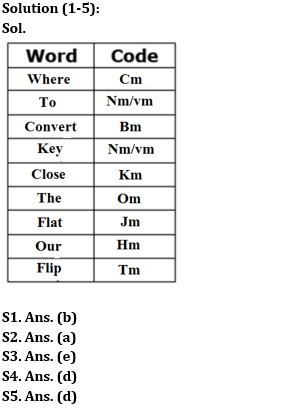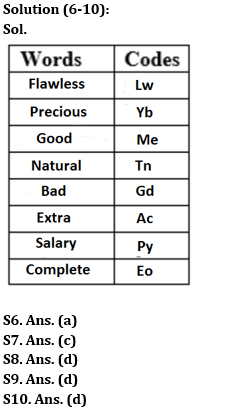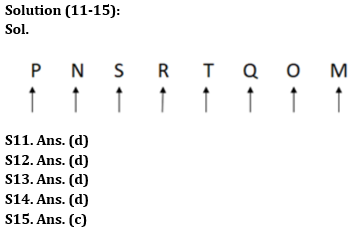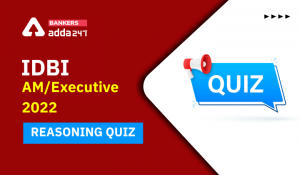Latest Banking jobs   »

# Reasoning Ability Quiz For SBI Clerk Prelims 2022- 30th May

Directions (1-5): Study the following information carefully and answer the given question.
In a certain code language,
‘Where to convert key’ is coded as ‘nm bm vm cm’
‘Close the flat’ is coded as ‘om km jm’
‘Convert our close’ is coded as ‘bm km hm’
‘Flat flip where’ is coded as ‘jm cm tm’

Q1. What is the code for ‘the’?
(a) km
(b) om
(c) jm
(d) cm
(e) Cannot be determined

Q2. What will be the code for ‘Key to flat’?
(a) nm vm jm
(b) nm om jm
(c) bm vm cm
(d) km jm hm
(e) Cannot be determined

Q3. What is the code for ‘Key close’?
(a) nm km
(b) cm km
(c) vm km
(d) jm hm
(e) Cannot be determined

Q4. Which word is coded as ‘hm cm km’?
(a) Where to convert
(b) Where the close
(c) The convert to
(d) Where our close
(e) Cannot be determined

Q5. What could be the code for ‘Convert far flip’ if ‘far’ is coded as ‘pm’?
(a) hm jm tm
(b) om im um
(c) km jm hm
(d) bm tm pm
(e) bm nm gm

Directions (6-10): Study the following information and answer the given questions.
In a certain code language,
“Flawless precious good” is coded as “lw me yb”
“Natural precious bad” is coded as “tn yb gd”
“Extra Flawless salary” is coded as “ac lw py”
“Salary bad complete” is coded as “gd eo py”

Q6. What is the code of “Flawless”?
(a) lw
(b) me
(c) py
(d) ac
(e) None of these

Q7. How is “Salary” written in the given code language?
(a) gd
(b) eo
(c) py
(d) Cannot be determined
(e) None of the above

Q8. What does “yb” mean?
(a) Natural
(b) Good
(d) Precious
(e) None of the above

Q9. How is “Natural” coded in the given code language?
(a) lw
(b) eo
(c) ac
(d) tn
(e) None of the above

Q10. How is “Good” coded?
(a) ac
(b) lw
(c) py
(d) me
(e) None of the above

Directions (11-15): Study the following information carefully and answer the questions accordingly.
There are eight persons – M, N, O, P, Q, R, S and T. All of them sit in a straight row in a show, facing north direction but not necessarily in the same order. O is not a neighbor of S who is immediate right of N. The number of persons between Q and M is same as the number of persons between P and S. Q sits second to the right of R who sits fourth from the left end. M sits at an extreme end of the row. T sits immediate left of Q. R sits three places away from P.

Q11. How many persons sit to the left of O?
(a) Five
(b) Two
(c) Four
(d) Six
(e) None of these

Q12. If N is related to R, T is related to O, then in the same way, which of the following is related to M?
(a) The one who sits between N and R
(b) The one who sits fourth to the right of S
(c) The one who sits fourth from the right end
(d) The one who sits second to the right of R
(e) None of these

Q13. What is the position of T with respect to N?
(a) Fourth to the left
(b) Fourth to the right
(c) Second to the left
(d) Third to the right
(e) None of these

Q14. Four among the following five are same in a certain manner and forms a group. Which among the following does not belong to the group?
(a) The one who sits third to the right of P
(b) The one who sits fourth to the left of O
(c) The one who sits just right of Q
(d) The one who sits just left of N
(e) The one who sits second to the left of R

Q15. Which of the following sits third to the left of the one who sits fourth from the right end?
(a) S
(b) P
(c) N
(d) R
(e) None of these

Solutions#### Congratulations!Incorrect details? Fill the form again here

•Reasoning Ability Quiz For IBPS RRB PO P...
•Reasoning Ability Quiz For IBPS RRB PO P...
•Reasoning Ability Quiz For IDBI AM/Execu...
•Reasoning Ability Quiz For IBPS RRB PO P...
•Reasoning Ability Quiz For IBPS RRB PO P...
•Reasoning Ability Quiz For IDBI AM/Execu...